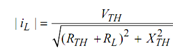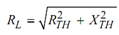## Maximum Power Transfer Theorem Assignment Help

Assignment Help: >> AC Circuits - Maximum Power Transfer Theorem

Maximum Power Transfer Theorem:

This theorem is utilized to determine the value of load, for which source shall transfer the maximum power. Before applying the maximum power transfer theorem, we apply Thevenin theorem across load terminals. Now there are following three cases for load to receive the maximum power.

Case I : Load is Pure Resistive

Now we have to determine load resistance RL so that maximum power is transferred to it.

iL  = VTH / (RTH + RL ) + j XTHPL = | iL |2 × RL = ((VTH) 2/ (R TH + R L) 2 + (X TH) 2) × RL

Condition for maximum power

dPL/ dRL    = 0

(V TH )2   = {(R TH + RL  )2 + (X TH )2 } - R L 2 (R TH + RL  ) / {(R Th + R L  )2 + (X TH) 2   } = 0

(RTH + RL  )2 + (XTH )2        = 2 RL (RTH + RL  )

⇒         (RL) 2 = (R TH) 2 + (X TH) 2

or,= | ZTH  |

So load resistance RL should be equal to the magnitude of the internal impedance of circuit.

 Load is Complex Maximum Power Transfer in Case of DC Network